# 二叉树¶

「二叉树 Binary Tree」是一种非线性数据结构，代表着祖先与后代之间的派生关系，体现着 “一分为二” 的分治逻辑。类似于链表，二叉树也是以结点为单位存储的，结点包含「值」和两个「指针」。

/* 链表结点类 */
class TreeNode {
int val;         // 结点值
TreeNode left;   // 左子结点指针
TreeNode right;  // 右子结点指针
TreeNode(int x) { val = x; }
}

/* 链表结点结构体 */
struct TreeNode {
int val;          // 结点值
TreeNode *left;   // 左子结点指针
TreeNode *right;  // 右子结点指针
TreeNode(int x) : val(x), left(nullptr), right(nullptr) {}
};

""" 链表结点类 """
class TreeNode:
def __init__(self, val=0, left=None, right=None):
self.val = val      # 结点值
self.left = left    # 左子结点指针
self.right = right  # 右子结点指针

""" 链表结点类 """
type TreeNode struct {
Val   int
Left  *TreeNode
Right *TreeNode
}
""" 结点初始化方法 """
func NewTreeNode(v int) *TreeNode {
return &TreeNode{
Left:  nil,
Right: nil,
Val:   v,
}
}

/* 链表结点类 */
function TreeNode(val, left, right) {
this.val = (val === undefined ? 0 : val); // 结点值
this.left = (left === undefined ? null : left); // 左子结点指针
this.right = (right === undefined ? null : right); // 右子结点指针
}

/* 链表结点类 */
class TreeNode {
val: number;
left: TreeNode | null;
right: TreeNode | null;

constructor(val?: number, left?: TreeNode | null, right?: TreeNode | null) {
this.val = val === undefined ? 0 : val; // 结点值
this.left = left === undefined ? null : left; // 左子结点指针
this.right = right === undefined ? null : right; // 右子结点指针
}
}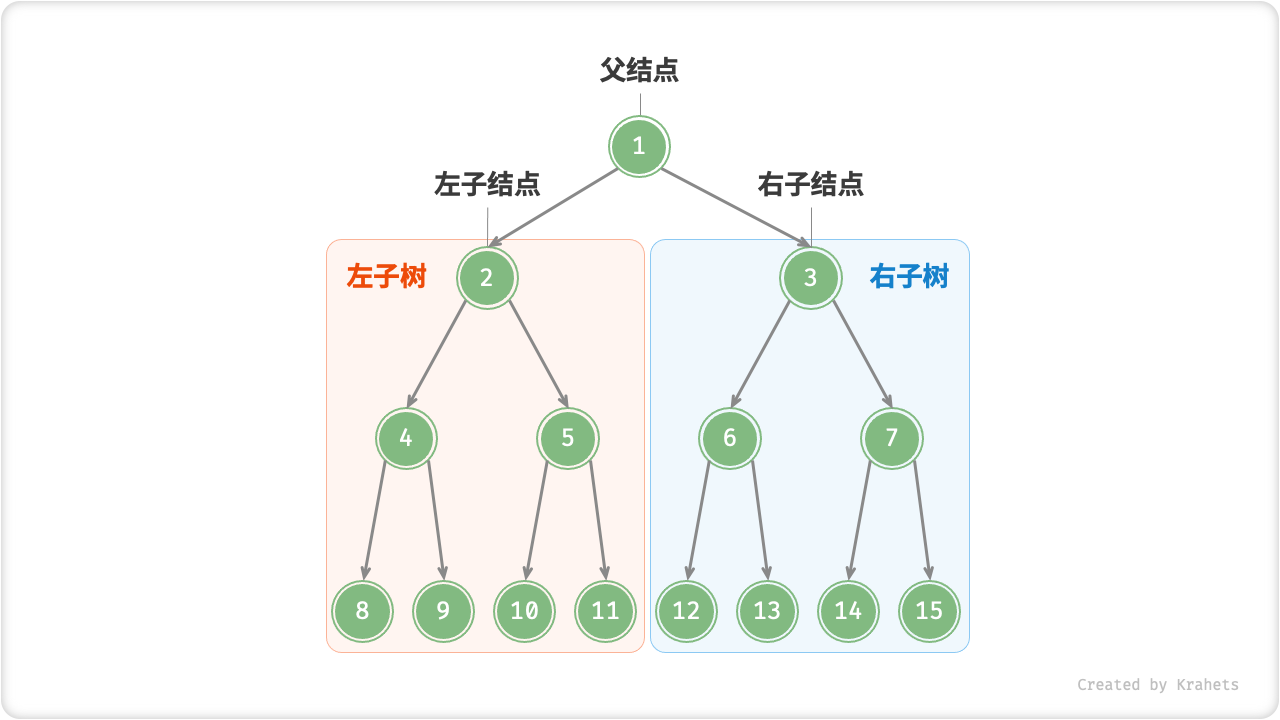Fig. 子结点与子树

## 二叉树常见术语¶

• 「根结点 Root Node」：二叉树最顶层的结点，其没有父结点；
• 「叶结点 Leaf Node」：没有子结点的结点，其两个指针都指向 $$\text{null}$$
• 结点所处「层 Level」：从顶置底依次增加，根结点所处层为 1 ；
• 结点「度 Degree」：结点的子结点数量，二叉树中度的范围是 0, 1, 2 ；
• 「边 Edge」：连接两个结点的边，即结点指针；
• 二叉树「高度」：二叉树中根结点到最远叶结点走过边的数量；
• 结点「深度 Depth」 ：根结点到该结点走过边的数量；
• 结点「高度 Height」：最远叶结点到该结点走过边的数量；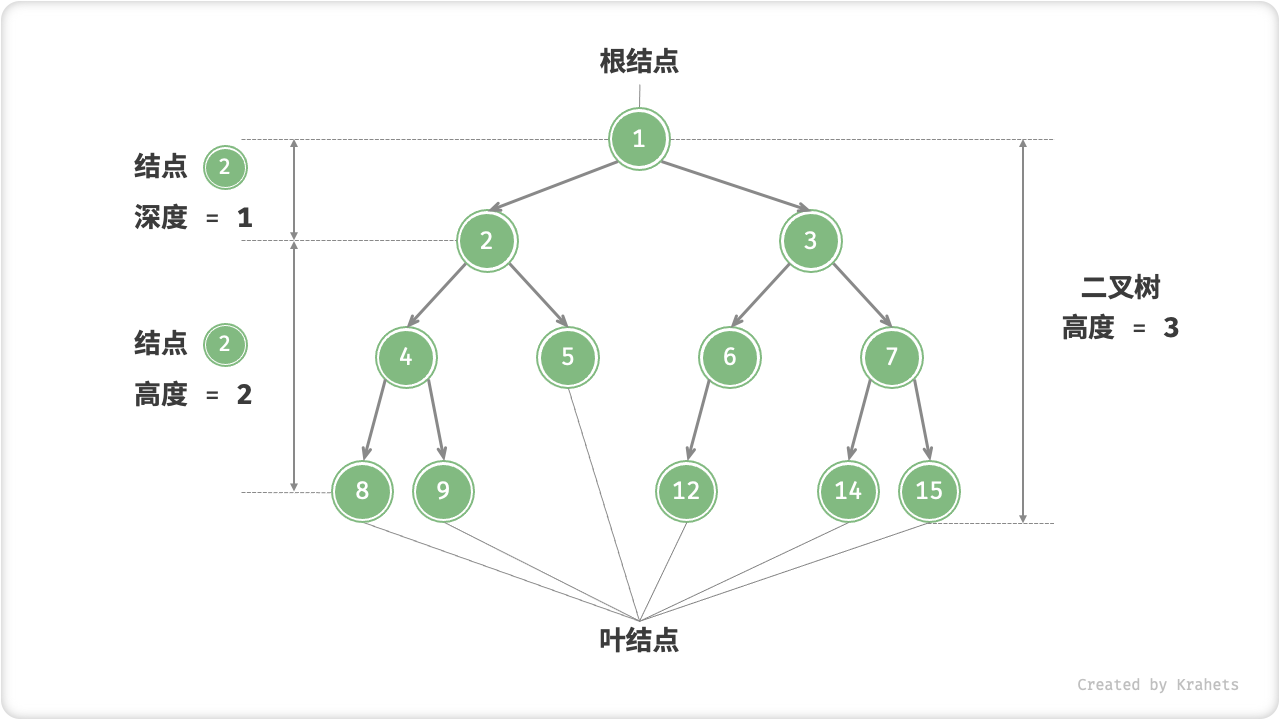Fig. 二叉树的常见术语

## 二叉树最佳和最差结构¶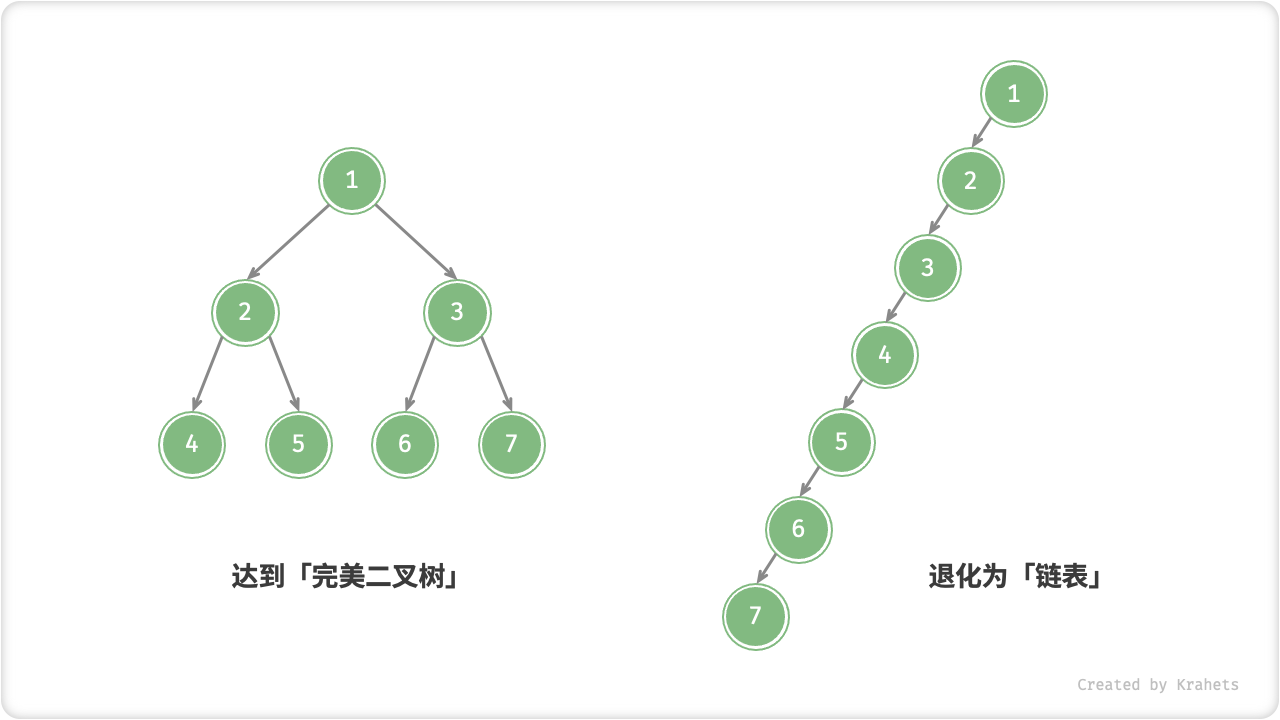Fig. 二叉树的最佳和最差结构

$$i$$ 层的结点数量 $$2^{i-1}$$ $$1$$

## 二叉树基本操作¶

binary_tree.java
// 初始化结点
TreeNode n1 = new TreeNode(1);
TreeNode n2 = new TreeNode(2);
TreeNode n3 = new TreeNode(3);
TreeNode n4 = new TreeNode(4);
TreeNode n5 = new TreeNode(5);
// 构建引用指向（即指针）
n1.left = n2;
n1.right = n3;
n2.left = n4;
n2.right = n5;

binary_tree.cpp
/* 初始化二叉树 */
// 初始化结点
TreeNode* n1 = new TreeNode(1);
TreeNode* n2 = new TreeNode(2);
TreeNode* n3 = new TreeNode(3);
TreeNode* n4 = new TreeNode(4);
TreeNode* n5 = new TreeNode(5);
// 构建引用指向（即指针）
n1->left = n2;
n1->right = n3;
n2->left = n4;
n2->right = n5;

binary_tree.py


binary_tree.go
/* 初始化二叉树 */
// 初始化结点
n1 := NewTreeNode(1)
n2 := NewTreeNode(2)
n3 := NewTreeNode(3)
n4 := NewTreeNode(4)
n5 := NewTreeNode(5)
// 构建引用指向（即指针）
n1.Left = n2
n1.Right = n3
n2.Left = n4
n2.Right = n5

binary_tree.js
/* 初始化二叉树 */
// 初始化结点
let n1 = new TreeNode(1),
n2 = new TreeNode(2),
n3 = new TreeNode(3),
n4 = new TreeNode(4),
n5 = new TreeNode(5);
// 构建引用指向（即指针）
n1.left = n2;
n1.right = n3;
n2.left = n4;
n2.right = n5;

binary_tree.ts
/* 初始化二叉树 */
// 初始化结点
let n1 = new TreeNode(1),
n2 = new TreeNode(2),
n3 = new TreeNode(3),
n4 = new TreeNode(4),
n5 = new TreeNode(5);
// 构建引用指向（即指针）
n1.left = n2;
n1.right = n3;
n2.left = n4;
n2.right = n5;

binary_tree.c


binary_tree.cs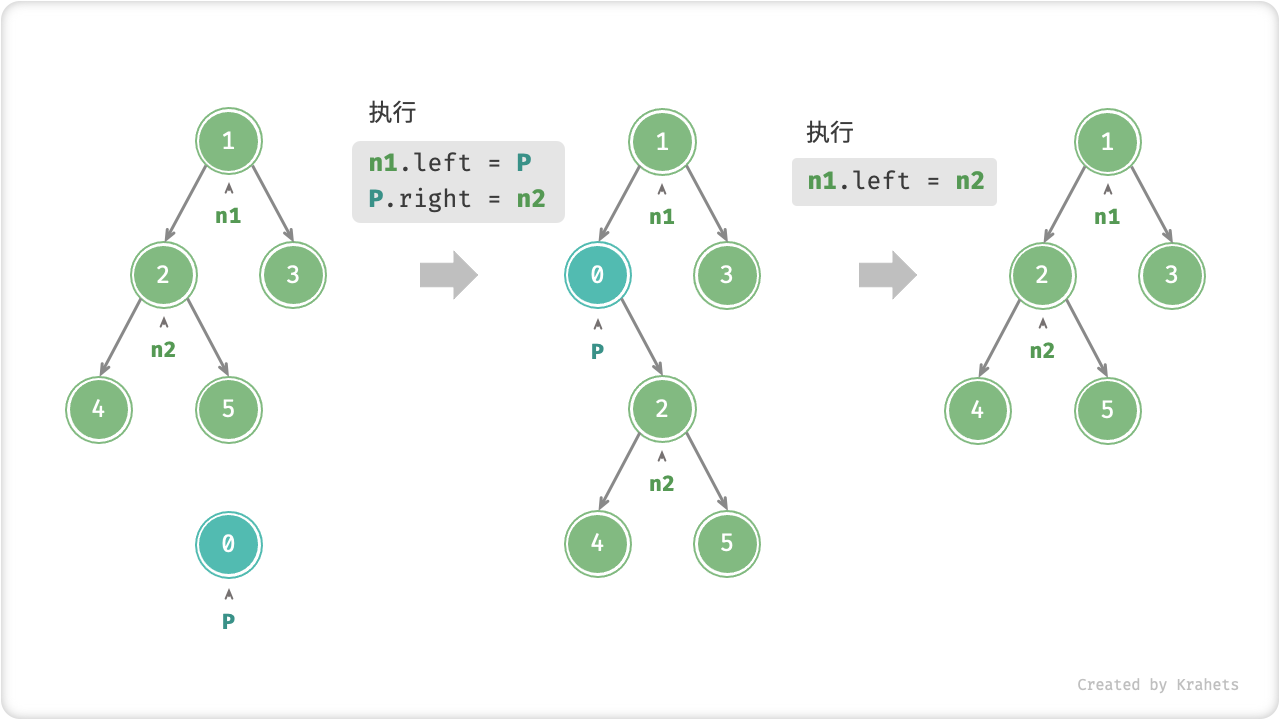Fig. 在二叉树中插入与删除结点

binary_tree.java
TreeNode P = new TreeNode(0);
// 在 n1 -> n2 中间插入结点 P
n1.left = P;
P.left = n2;
// 删除结点 P
n1.left = n2;

binary_tree.cpp
/* 插入与删除结点 */
TreeNode* P = new TreeNode(0);
// 在 n1 -> n2 中间插入结点 P
n1->left = P;
P->left = n2;
// 删除结点 P
n1->left = n2;

binary_tree.py


binary_tree.go
/* 插入与删除结点 */
// 在 n1 -> n2 中间插入结点 P
p := NewTreeNode(0)
n1.Left = p
p.Left = n2
// 删除结点 P
n1.Left = n2

binary_tree.js
/* 插入与删除结点 */
let P = new TreeNode(0);
// 在 n1 -> n2 中间插入结点 P
n1.left = P;
P.left = n2;
// 删除结点 P
n1.left = n2;

binary_tree.ts
/* 插入与删除结点 */
const P = new TreeNode(0);
// 在 n1 -> n2 中间插入结点 P
n1.left = P;
P.left = n2;
// 删除结点 P
n1.left = n2;

binary_tree.c


binary_tree.cs



Note

## 二叉树遍历¶

### 层序遍历¶

「层序遍历 Hierarchical-Order Traversal」从顶至底、一层一层地遍历二叉树，并在每层中按照从左到右的顺序访问结点。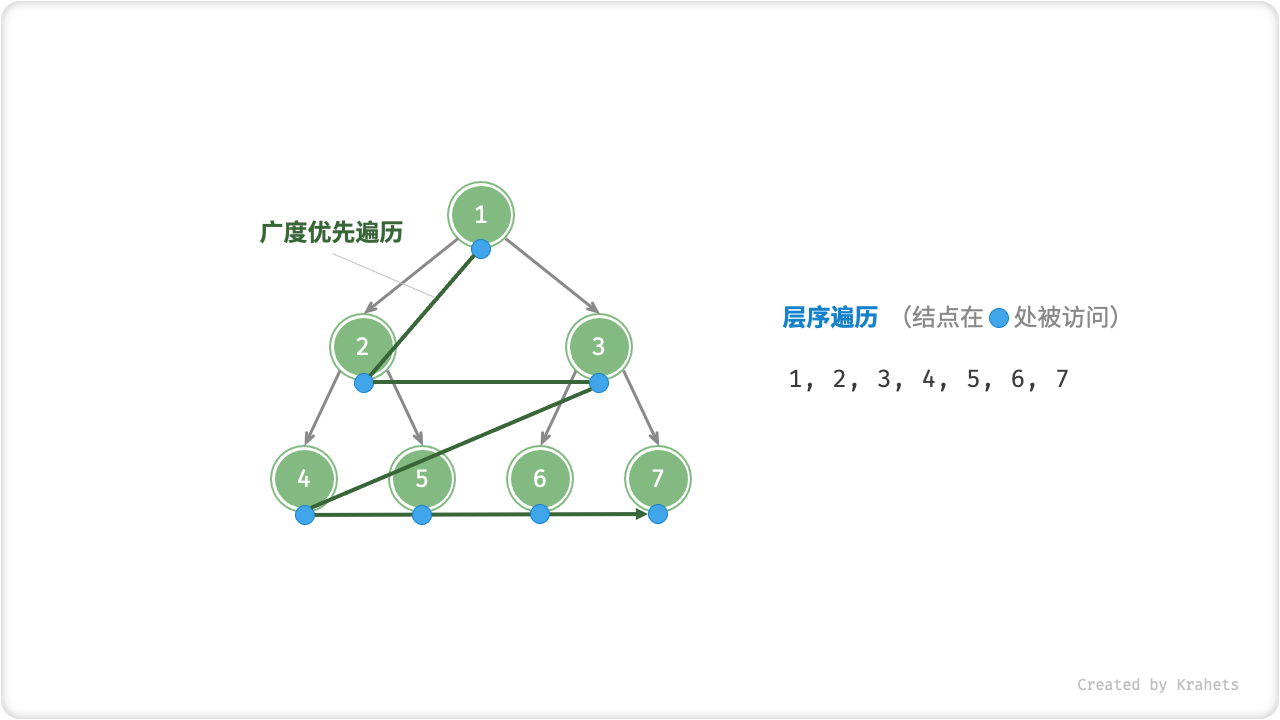Fig. 二叉树的层序遍历

binary_tree_bfs.java
/* 层序遍历 */
List<Integer> hierOrder(TreeNode root) {
// 初始化队列，加入根结点
// 初始化一个列表，用于保存遍历序列
List<Integer> list = new ArrayList<>();
while (!queue.isEmpty()) {
TreeNode node = queue.poll();  // 队列出队
if (node.left != null)
queue.offer(node.left);    // 左子结点入队
if (node.right != null)
queue.offer(node.right);   // 右子结点入队
}
return list;
}

binary_tree_bfs.cpp
/* 层序遍历 */
vector<int> hierOrder(TreeNode* root) {
// 初始化队列，加入根结点
queue<TreeNode*> queue;
queue.push(root);
// 初始化一个列表，用于保存遍历序列
vector<int> vec;
while (!queue.empty()) {
TreeNode* node = queue.front();
queue.pop();  // 队列出队
vec.push_back(node->val);            // 保存结点
if (node->left != nullptr)
queue.push(node->left);    // 左子结点入队
if (node->right != nullptr)
queue.push(node->right);   // 右子结点入队
}
return vec;
}

binary_tree_bfs.py


binary_tree_bfs.go
/* 层序遍历 */
func levelOrder(root *TreeNode) []int {
// 初始化队列，加入根结点
queue := list.New()
queue.PushBack(root)
// 初始化一个切片，用于保存遍历序列
nums := make([]int, 0)
for queue.Len() > 0 {
// poll
node := queue.Remove(queue.Front()).(*TreeNode)
// 保存结点
nums = append(nums, node.Val)
if node.Left != nil {
// 左子结点入队
queue.PushBack(node.Left)
}
if node.Right != nil {
// 右子结点入队
queue.PushBack(node.Right)
}
}
return nums
}

binary_tree_bfs.js
/* 层序遍历 */
function hierOrder(root) {
// 初始化队列，加入根结点
let queue = [root];
// 初始化一个列表，用于保存遍历序列
let list = [];
while (queue.length) {
let node = queue.shift();  // 队列出队
list.push(node.val);          // 保存结点
if (node.left)
queue.push(node.left);    // 左子结点入队
if (node.right)
queue.push(node.right);   // 右子结点入队
}
return list;
}

binary_tree_bfs.ts
/* 层序遍历 */
function hierOrder(root: TreeNode | null): number[] {
// 初始化队列，加入根结点
const queue = [root];
// 初始化一个列表，用于保存遍历序列
const list: number[] = [];
while (queue.length) {
let node = queue.shift() as TreeNode; // 队列出队
list.push(node.val); // 保存结点
if (node.left) {
queue.push(node.left); // 左子结点入队
}
if (node.right) {
queue.push(node.right); // 右子结点入队
}
}
return list;
}

binary_tree_bfs.c


binary_tree_bfs.cs



### 前序、中序、后序遍历¶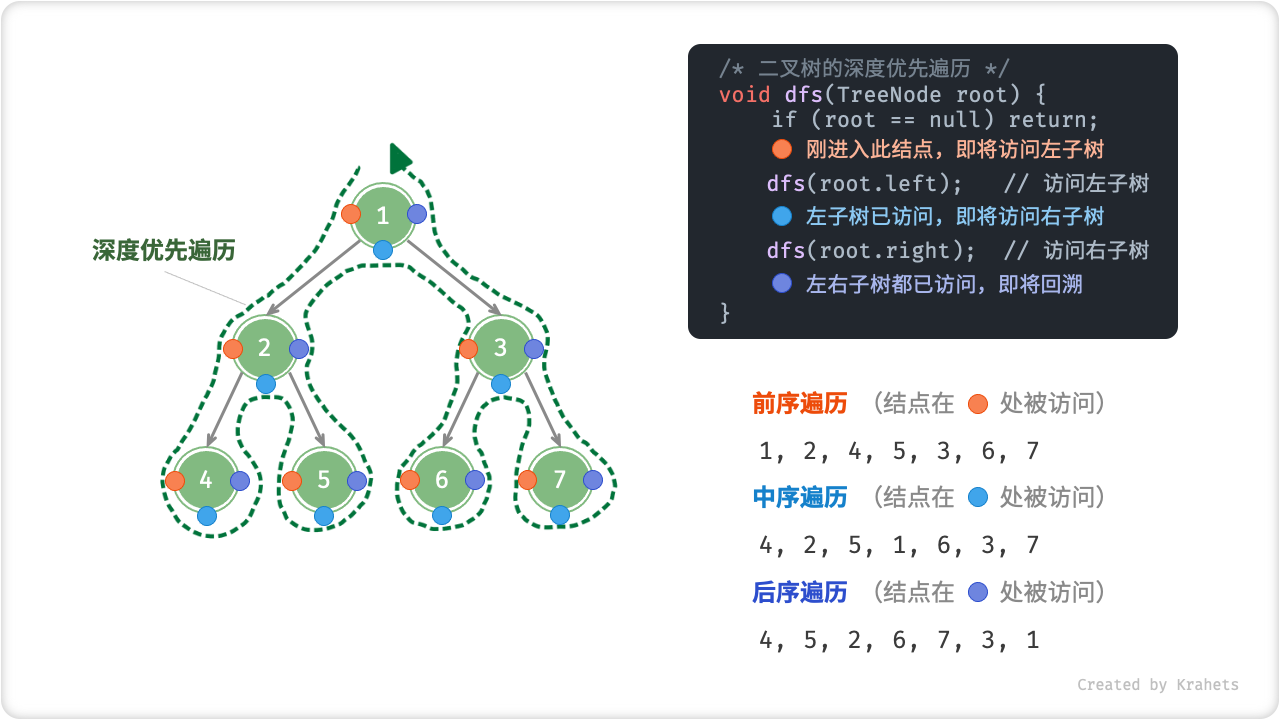Fig. 二叉树的前 / 中 / 后序遍历

binary_tree_dfs.java
/* 前序遍历 */
void preOrder(TreeNode root) {
if (root == null) return;
// 访问优先级：根结点 -> 左子树 -> 右子树
preOrder(root.left);
preOrder(root.right);
}

/* 中序遍历 */
void inOrder(TreeNode root) {
if (root == null) return;
// 访问优先级：左子树 -> 根结点 -> 右子树
inOrder(root.left);
inOrder(root.right);
}

/* 后序遍历 */
void postOrder(TreeNode root) {
if (root == null) return;
// 访问优先级：左子树 -> 右子树 -> 根结点
postOrder(root.left);
postOrder(root.right);
}

binary_tree_dfs.cpp
/* 前序遍历 */
void preOrder(TreeNode* root) {
if (root == nullptr) return;
// 访问优先级：根结点 -> 左子树 -> 右子树
vec.push_back(root->val);
preOrder(root->left);
preOrder(root->right);
}

/* 中序遍历 */
void inOrder(TreeNode* root) {
if (root == nullptr) return;
// 访问优先级：左子树 -> 根结点 -> 右子树
inOrder(root->left);
vec.push_back(root->val);
inOrder(root->right);
}

/* 后序遍历 */
void postOrder(TreeNode* root) {
if (root == nullptr) return;
// 访问优先级：左子树 -> 右子树 -> 根结点
postOrder(root->left);
postOrder(root->right);
vec.push_back(root->val);
}

binary_tree_dfs.py


binary_tree_dfs.go
/* 前序遍历 */
func preOrder(node *TreeNode) {
if node == nil {
return
}
// 访问优先级：根结点 -> 左子树 -> 右子树
nums = append(nums, node.Val)
preOrder(node.Left)
preOrder(node.Right)
}

/* 中序遍历 */
func inOrder(node *TreeNode) {
if node == nil {
return
}
// 访问优先级：左子树 -> 根结点 -> 右子树
inOrder(node.Left)
nums = append(nums, node.Val)
inOrder(node.Right)
}

/* 后序遍历 */
func postOrder(node *TreeNode) {
if node == nil {
return
}
// 访问优先级：左子树 -> 右子树 -> 根结点
postOrder(node.Left)
postOrder(node.Right)
nums = append(nums, node.Val)
}

binary_tree_dfs.js
/* 前序遍历 */
function preOrder(root){
if (root === null) return;
// 访问优先级：根结点 -> 左子树 -> 右子树
list.push(root.val);
preOrder(root.left);
preOrder(root.right);
}

/* 中序遍历 */
function inOrder(root) {
if (root === null) return;
// 访问优先级：左子树 -> 根结点 -> 右子树
inOrder(root.left);
list.push(root.val);
inOrder(root.right);
}

/* 后序遍历 */
function postOrder(root) {
if (root === null) return;
// 访问优先级：左子树 -> 右子树 -> 根结点
postOrder(root.left);
postOrder(root.right);
list.push(root.val);
}

binary_tree_dfs.ts
/* 前序遍历 */
function preOrder(root: TreeNode | null): void {
if (root === null) {
return;
}
// 访问优先级：根结点 -> 左子树 -> 右子树
list.push(root.val);
preOrder(root.left);
preOrder(root.right);
}

/* 中序遍历 */
function inOrder(root: TreeNode | null): void {
if (root === null) {
return;
}
// 访问优先级：左子树 -> 根结点 -> 右子树
inOrder(root.left);
list.push(root.val);
inOrder(root.right);
}

/* 后序遍历 */
function postOrder(root: TreeNode | null): void {
if (root === null) {
return;
}
// 访问优先级：左子树 -> 右子树 -> 根结点
postOrder(root.left);
postOrder(root.right);
list.push(root.val);
}

binary_tree_dfs.c


binary_tree_dfs.cs



Note